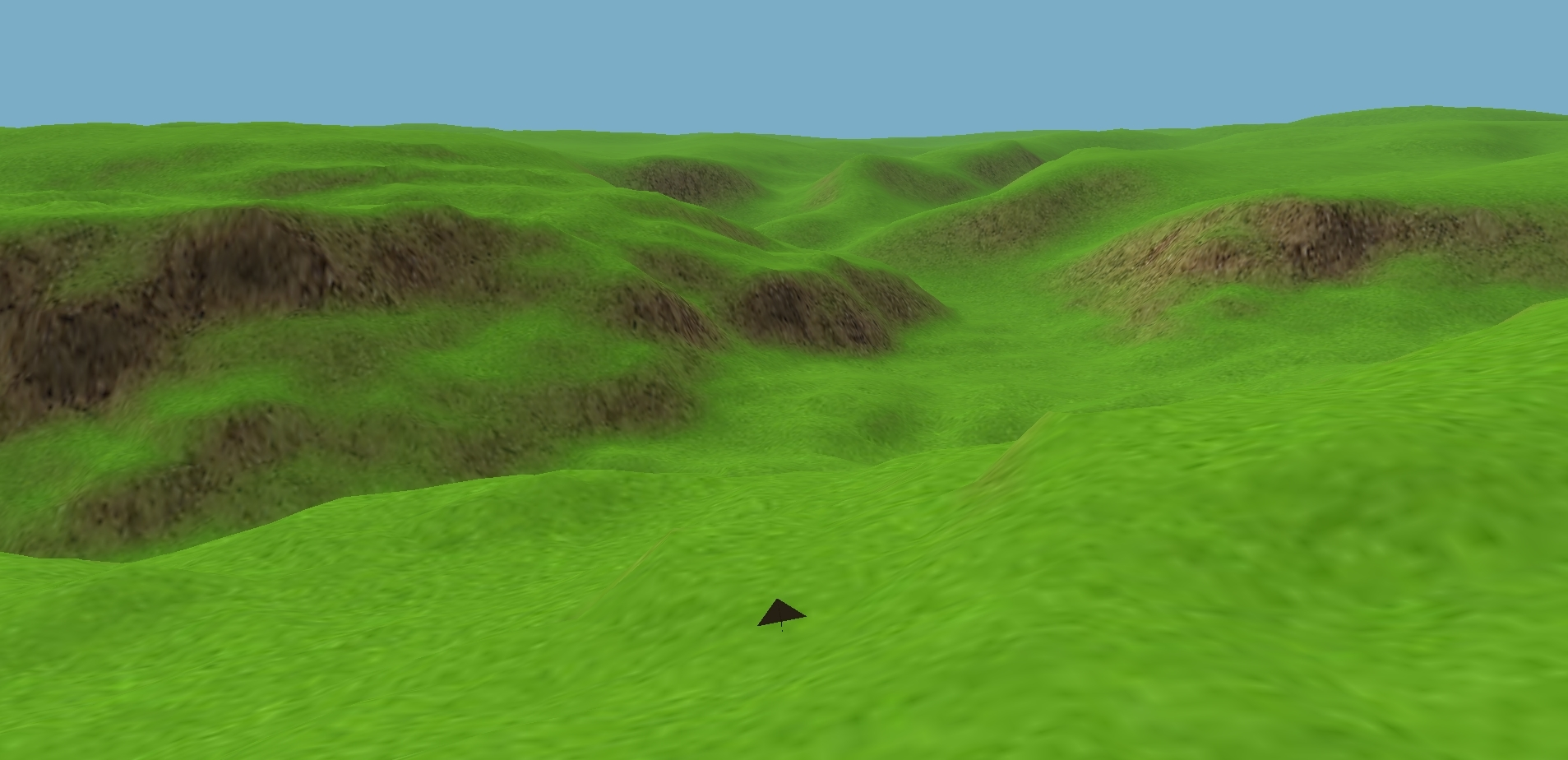# Algorithm for "mipmapping" terrain vertex heights and normals

I am creating an engine in opengl. As the distance from the player increases, the width and height of the tiles increase by factors of 2. How can I do this? I am currently just averaging, but I miss some of the peaks and valleys, so the slopes are less intense as the tile size increases. This causes some of the textures relative to slope angle to disappear (e.g. grass isn't formed on large slopes, but at distance everything becomes grassy).

The slopes/textures and lighting are based on my vertex normal map.

Here is pseudo code for my current procedure:

float heightmap[layer][x][y]
float normalmap[layer][x][y]

For i=1; i<num_mipmaps; i++
For x=0; x<(width>>i); x++
For y=0; y<(height>>i); y++
heightmap[i][x][y] = 0;
For x2=x*2-1; x2<x*2+1; x2++
For y2=y*2-1; y2<y*2+1; y2++
heightmap[i][x][y] += heightmap[i-1][x2][y2];
heightmap[i][x][y] /= 9;


... then I just calculate all face normals and vertex normals separately for each layer.• Do you mean LOD? Jan 17, 2018 at 16:51
• Yes, thank you. I have been looking for this term! Jan 17, 2018 at 17:15